﻿ 基于LSD与统计分析的航拍图像电力线提取方法
«上一篇文章快速检索 高级检索

 应用科技2019, Vol. 46Issue (2): 30-34  DOI: 10.11991/yykj.2018110010

### 引用本文LIN Huoduan, CHEN Jie, XUE Huachun, et al. Segmenting power lines on the aerial photos based on line segment detector and statistical analysis[J]. Applied Science and Technology, 2019, 46(2), 30-34. DOI: 10.11991/yykj.201811001.### 文章历史

1. 国网福建省电力有限公司 漳州公司，福建 漳州 363000;
2. 河海大学 物联网工程学院，江苏 常州 213022

Segmenting power lines on the aerial photos based on line segment detector and statistical analysis
LIN Huoduan1, CHEN Jie1, XUE Huachun1, XU Chang2, MA Yunpeng21. State Grid Fujian Provincial Electric Power Supply Company, Zhangzhou Branch, Zhangzhou 363000, China;
2. College of Internet of Things Engineering, Hohai University, Changzhou 213022, China
Abstract: In order to solve the problems of low accuracy and poor stability existing in power line extraction algorithms under complex scenes and different forms of power line environment, a new power line extraction method based on line segment detector (LSD) and statistical analysis is proposed in this paper. First, LSD algorithm was used to extract image line primitives; then the main direction of power line was determined according to statistical analysis, and the interference lines were removed from the main direction and by this way, a pool of power line primitives was constructed; second, the power line primitives were grouped and filtered by measuring the lateral distance to determine the power line primitives; finally, the extraction of power lines was determined by simulating each group of power line primitives. The experimental results show that the algorithm can effectively suppress the interference of aerial image background, and can detect both straight line and catenary power lines at the same time, having the advantages of high accuracy and good stability
Keywords: power lines extraction    catenary    LSD    statistical analysis    line primitives    main direction    least squares fitting    aerial image

1 电力线检测方法 1.1 算法流程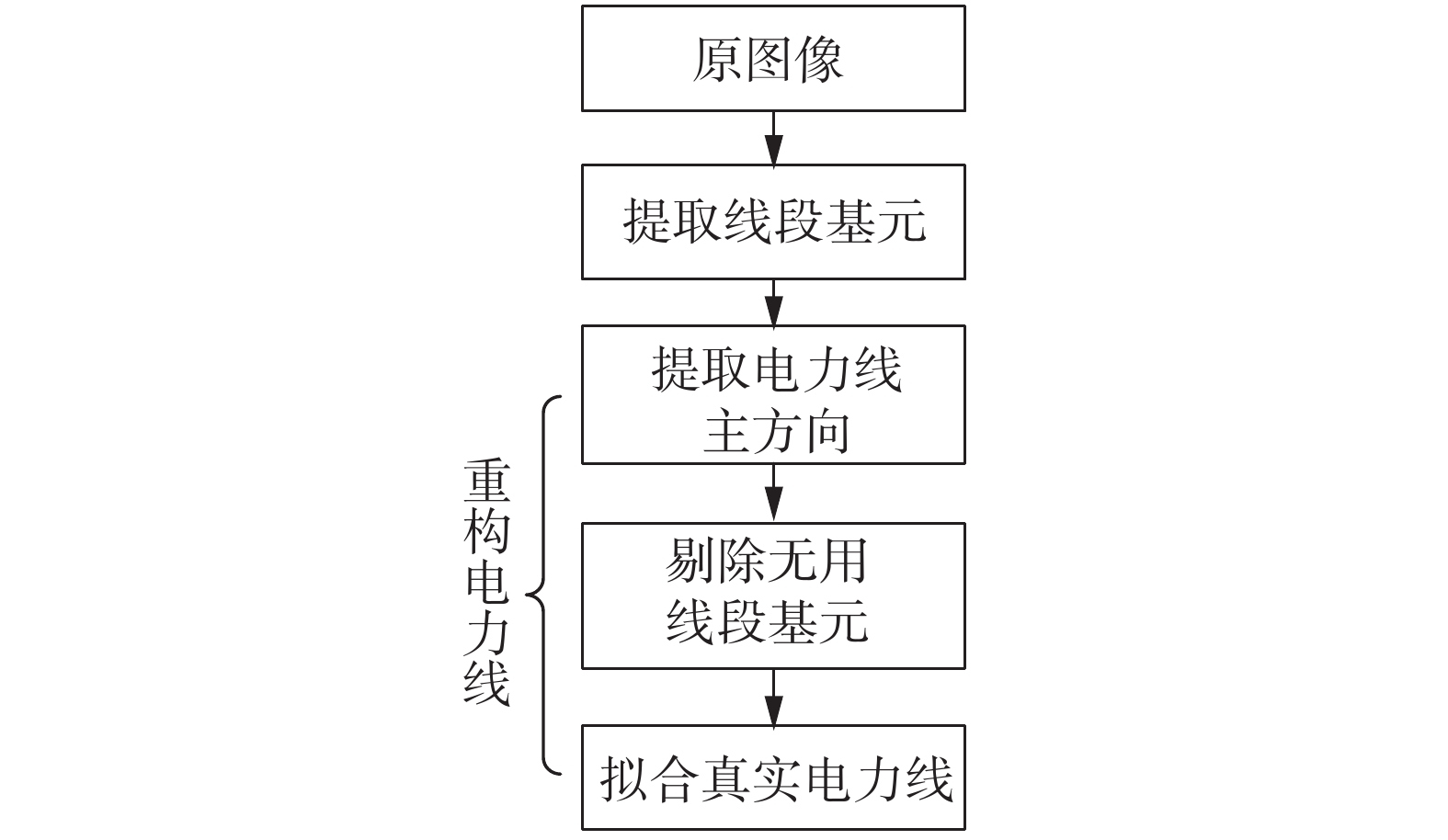Download: 图 1 算法执行过程
1.2 局部线段基元提取——LSD直线提取算法

LSD涉及到2个基本概念：梯度和图像的基准线(level-line)，如图2所示。LSD先计算每个像素与基准线的夹角以构建基准线场(level-line field)；然后利用区域生长算法合并场里方向近似一致的像素，得到一系列线支撑域(line support regions)，如图3所示；最后在这些域内进行像素合并提取直线段，并基于“Contrario model”和“Helmholtz principle”进行误差控制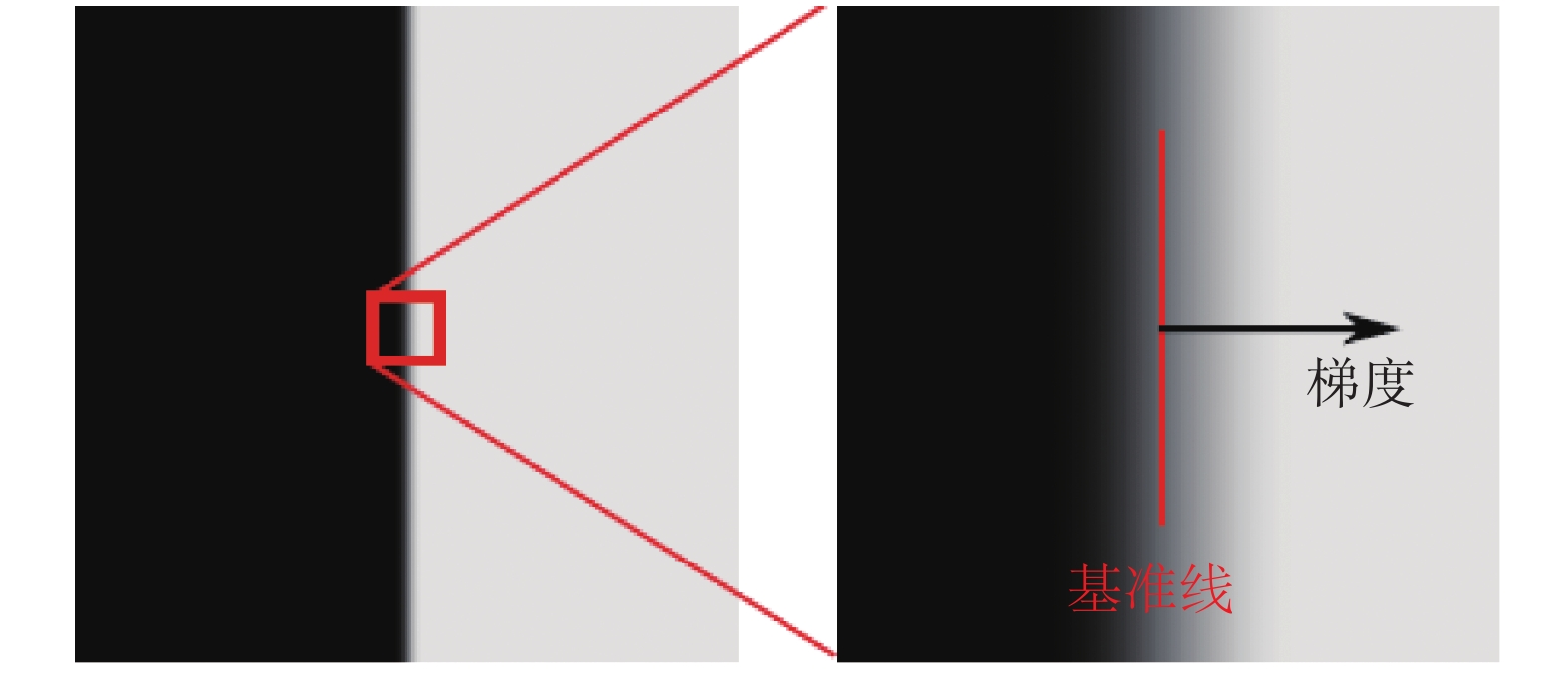Download: 图 2 图像梯度和基准线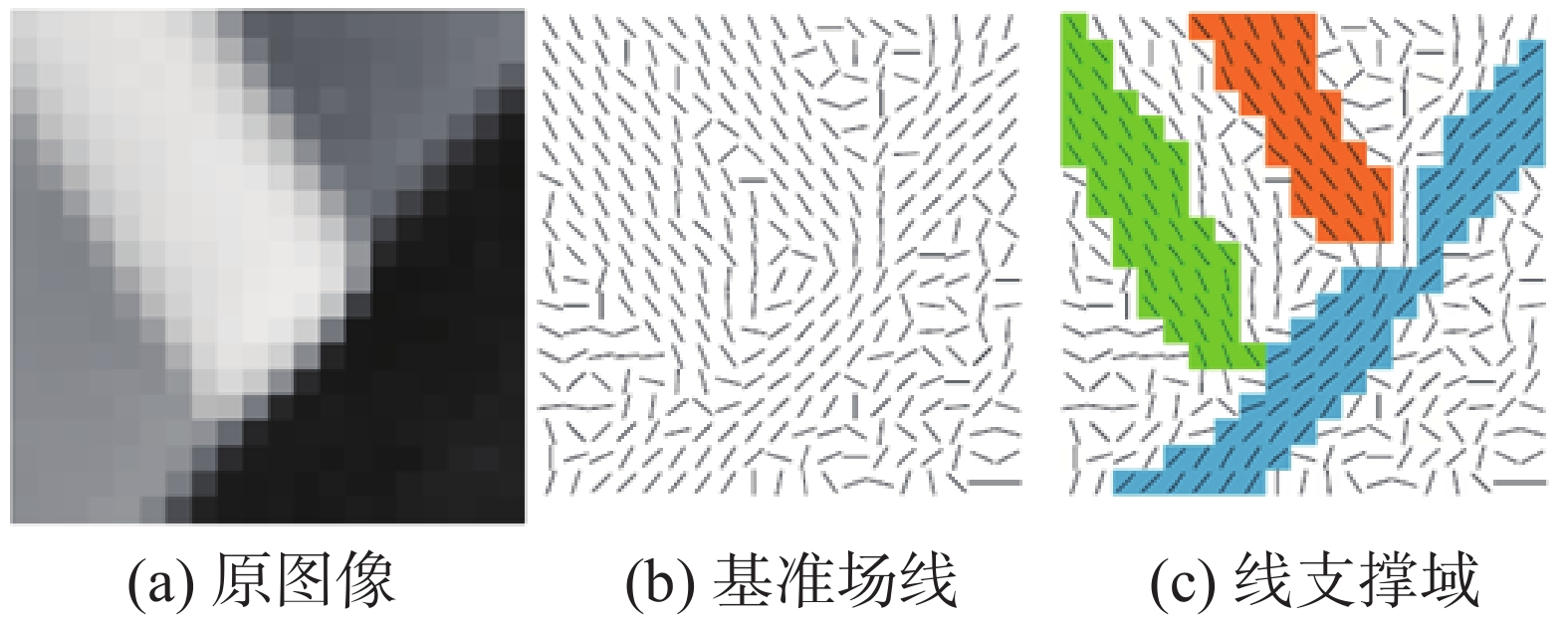Download: 图 3 线支撑域示意

LSD算法流程如图4所示，经过LSD直线提取算法处理，可得所有检测出线段基元的左右端点及线段宽度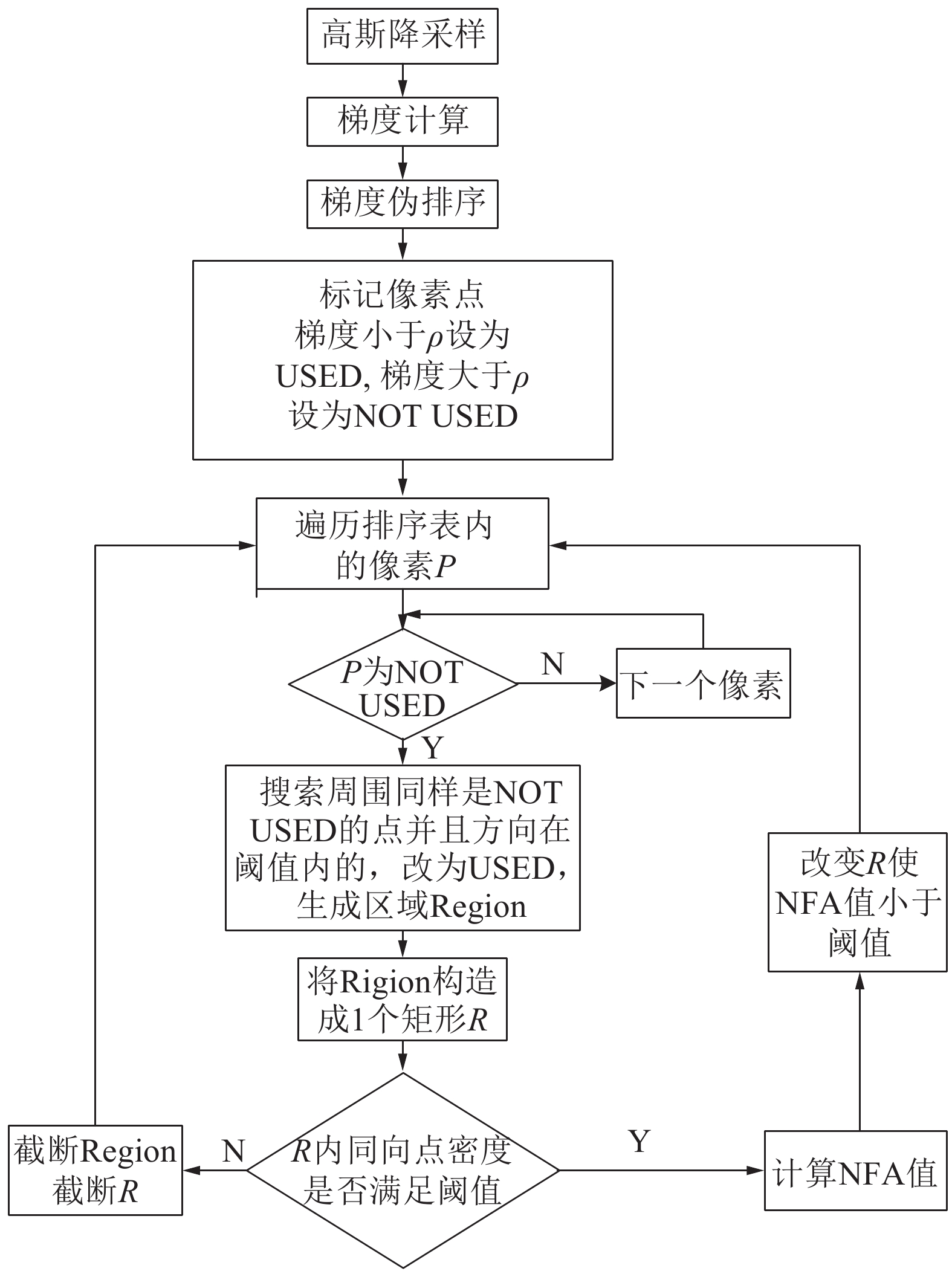Download: 图 4 LSD直线提取算法流程

1.3 中层视觉感知聚集——统计学特征 1.3.1 主方向的提取

1.3.2 基于侧向距离的线段基元的分析

 $\frac{{x - {a_1}}}{{{a_2} - {a_1}}} = \frac{{y - {b_1}}}{{{b_2} - {b_1}}},\frac{{x - {a_3}}}{{{a_4} - {a_3}}} = \frac{{y - {b_3}}}{{{b_4} - {b_3}}}$

 \begin{aligned} & \frac{{{b_2} - {b_1}}}{{{a_2} - {a_1}}}x - y - \frac{{{a_1} \times {b_2} - {a_2} \times {b_1}}}{{{a_2} - {a_1}}} = 0\\ & \frac{{{b_4} - {b_3}}}{{{a_4} - {a_3}}}x - y - \frac{{{a_3} \times {b_4} - {a_4} \times {b_3}}}{{{a_4} - {a_3}}} = 0 \end{aligned}

 \begin{aligned} & {D_1} = \left| {\frac{{{A_2} \times \displaystyle\frac{{\left( {{a_1} + {a_2}} \right)}}{2} + {B_2} \times \frac{{\left( {{b_1} + {b_2}} \right)}}{2} + {C_2}}}{{\sqrt {A_2^2 + B_2^2} }}} \right| = \\ & \left| {\frac{{\displaystyle\frac{{{b_4} - {b_3}}}{{{a_4} - {a_3}}} \times \frac{{\left( {{a_1} + {a_2}} \right)}}{2} + \left( { - 1} \right) \times \frac{{\left( {{b_1} + {b_2}} \right)}}{2} + \frac{{{a_3} \times {b_4} - {a_4} \times {b_3}}}{{{a_4} - {a_3}}}}}{{\sqrt {{{\left( {\displaystyle\frac{{{b_4} - {b_3}}}{{{a_4} - {a_3}}}} \right)}^2} + 1} }}} \right| \end{aligned} (1)

1.3.3 最小二乘法拟合

 $y = {\kern 1pt} {\sum\nolimits_{i = 0}^n a _i}{x^i} = {a_n}{x^n} + {a_{n - 1}}{x^{n - 1}} + \cdots + {a_1}x + {a_0}$

 ${{{X_0}}} = \left[ {\begin{array}{*{20}{c}} {x_1^n}&{x_1^{n - 1}}&\cdots&{x_1^2}&{{x_1}}&1\\ {x_2^n}&{x_2^{n - 1}}& \cdots &{x_2^2}&{x_2^{}}&1\\ \vdots & \cdots & \cdots &\cdots & \cdots &\vdots \\ {x_k^n}&{x_k^{n - 1}}& \cdots &{x_k^2}&{x_k^{}}&1 \end{array}} \right]$ (2)

 ${{A}} = {\left[ {{a_n},{a_{n - 1}}, \cdots ,{a_2},{a_1},{a_0}} \right]^{\rm{T}}}$

2 实验结果和分析

2.1 定性分析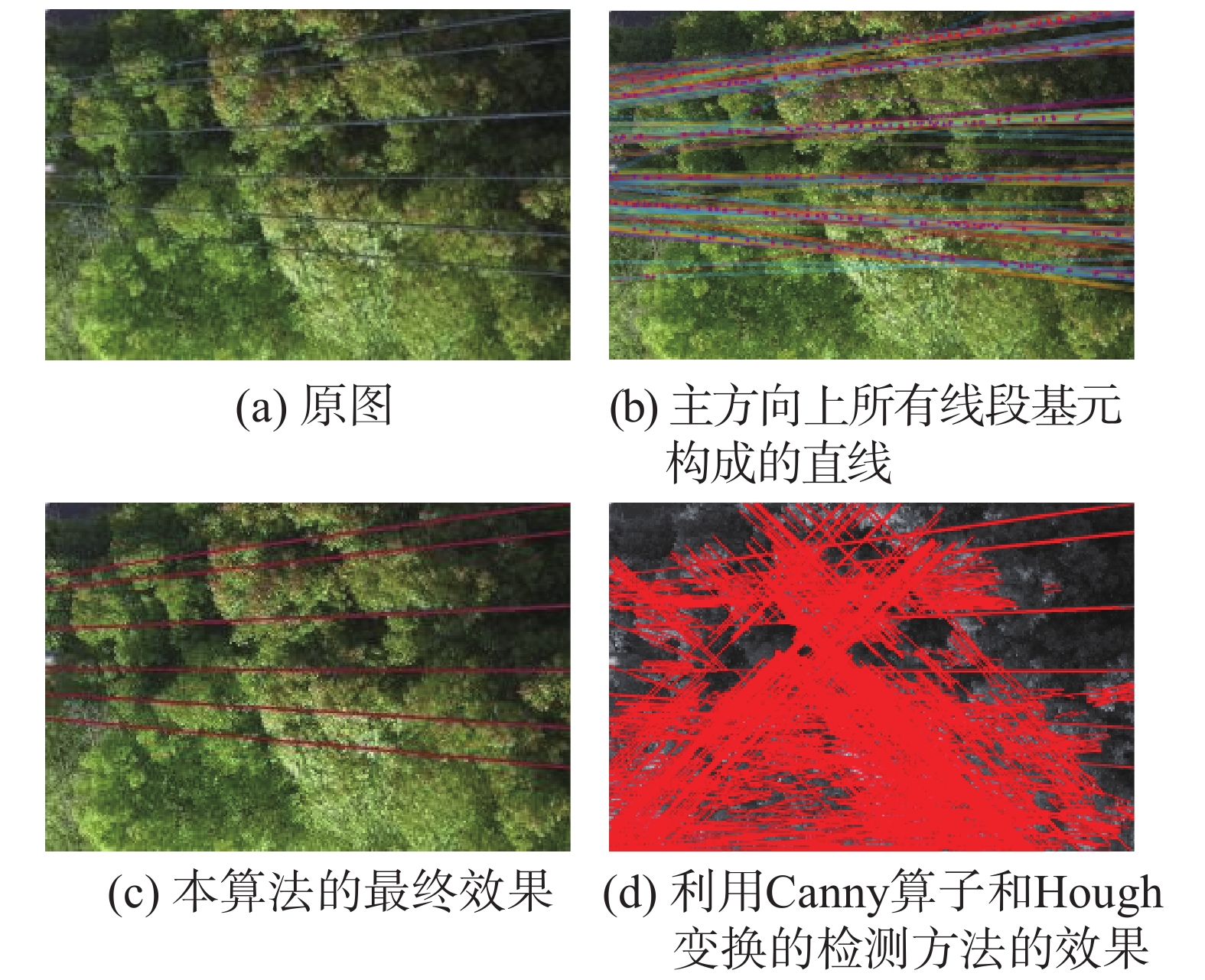Download: 图 5 电力线上方垂直下拍的电力线检测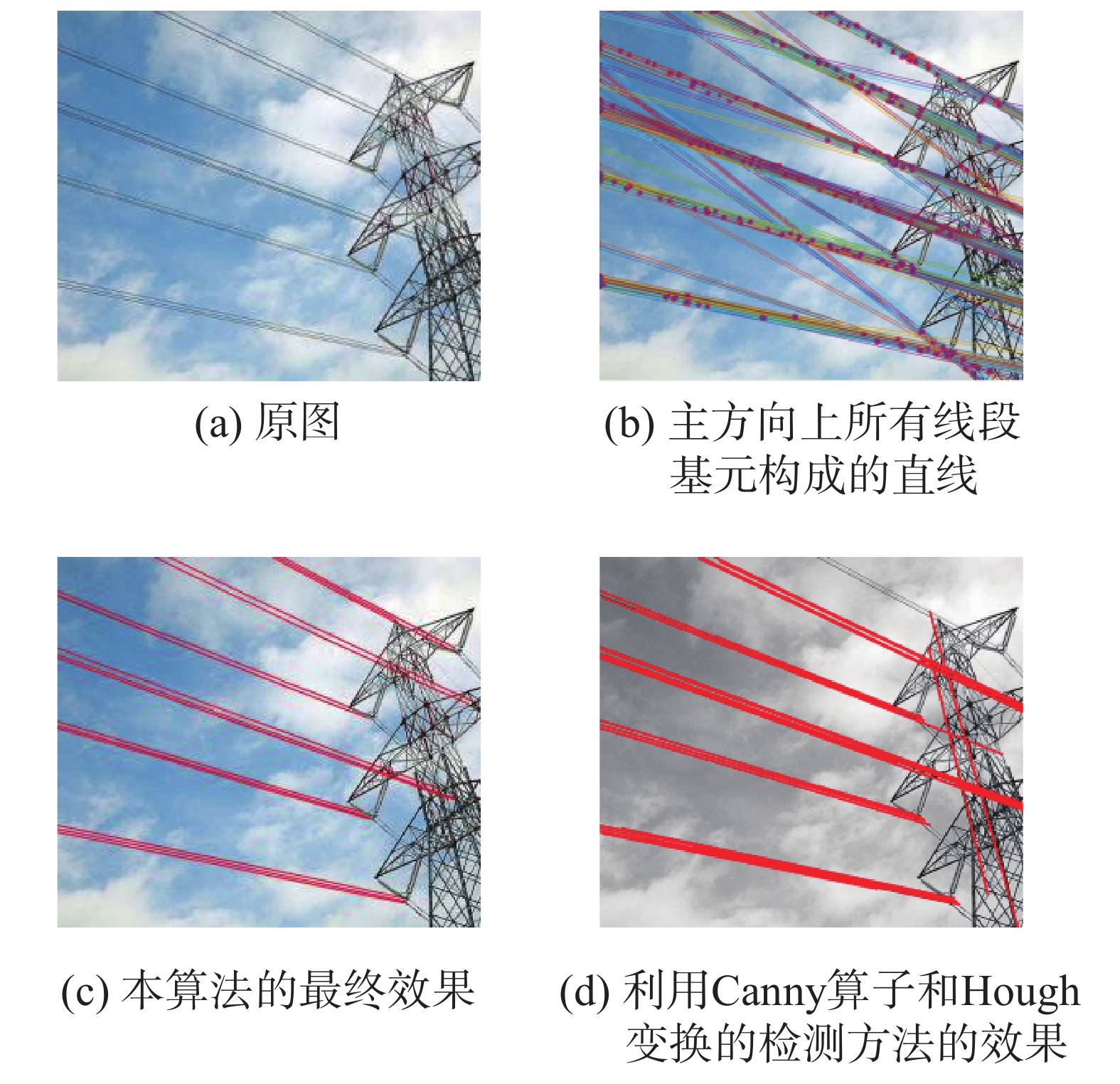Download: 图 6 电力公司提供的山区的电力线航拍图1检测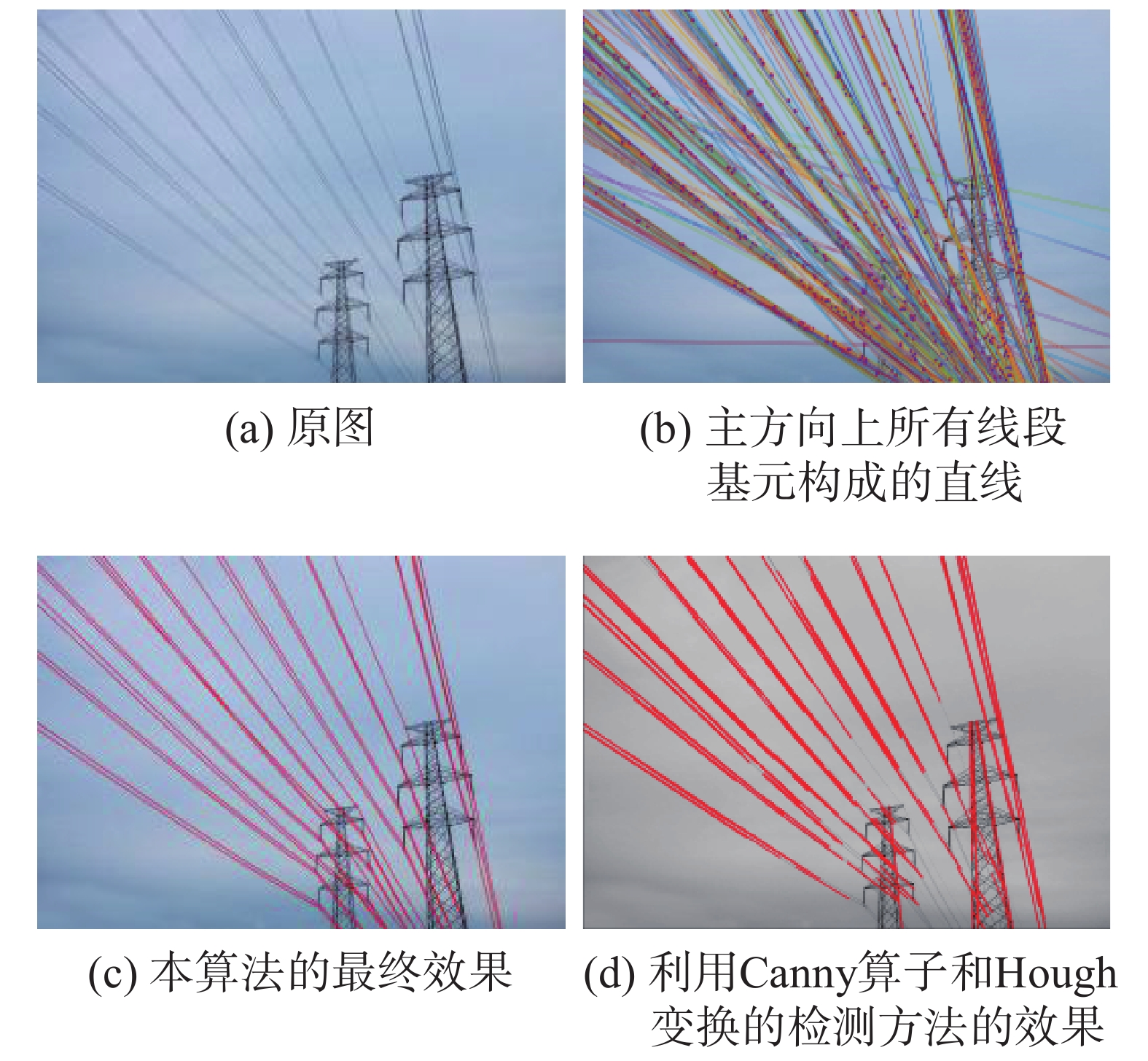Download: 图 7 电力公司提供的山区的电力线航拍图2检测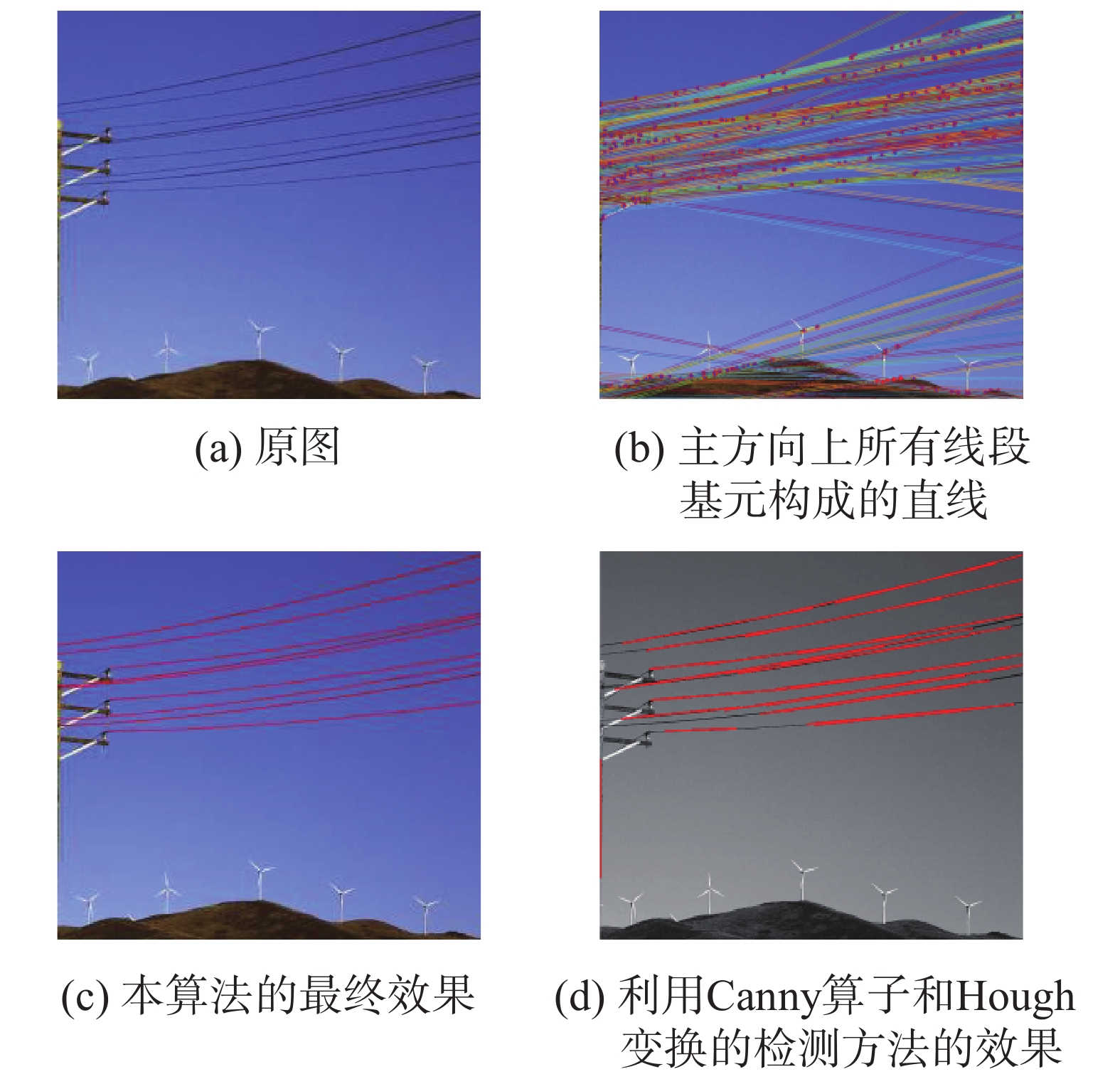Download: 图 8 电力公司提供的山区的电力线航拍图3检测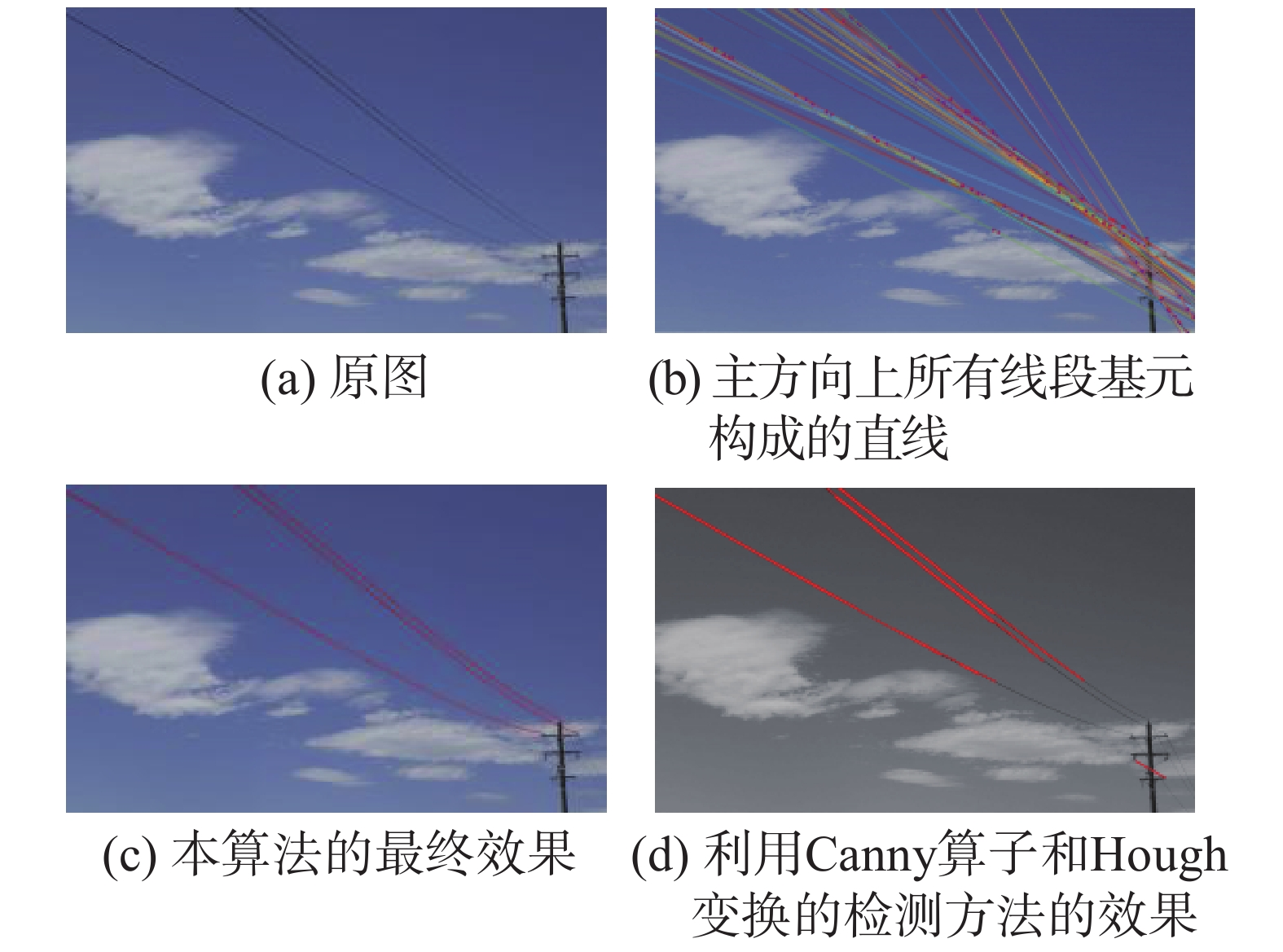Download: 图 9 电力公司提供的山区的电力线航拍图4检测
2.2 定量分析

 ${R_C} = \frac{{{N_{{\rm{correct}}}}}}{{{N_{{\rm{all}}}}}},{R_E} = \frac{{{N_{{\rm{error}}}}}}{{{N_{{\rm{all}}}}}}$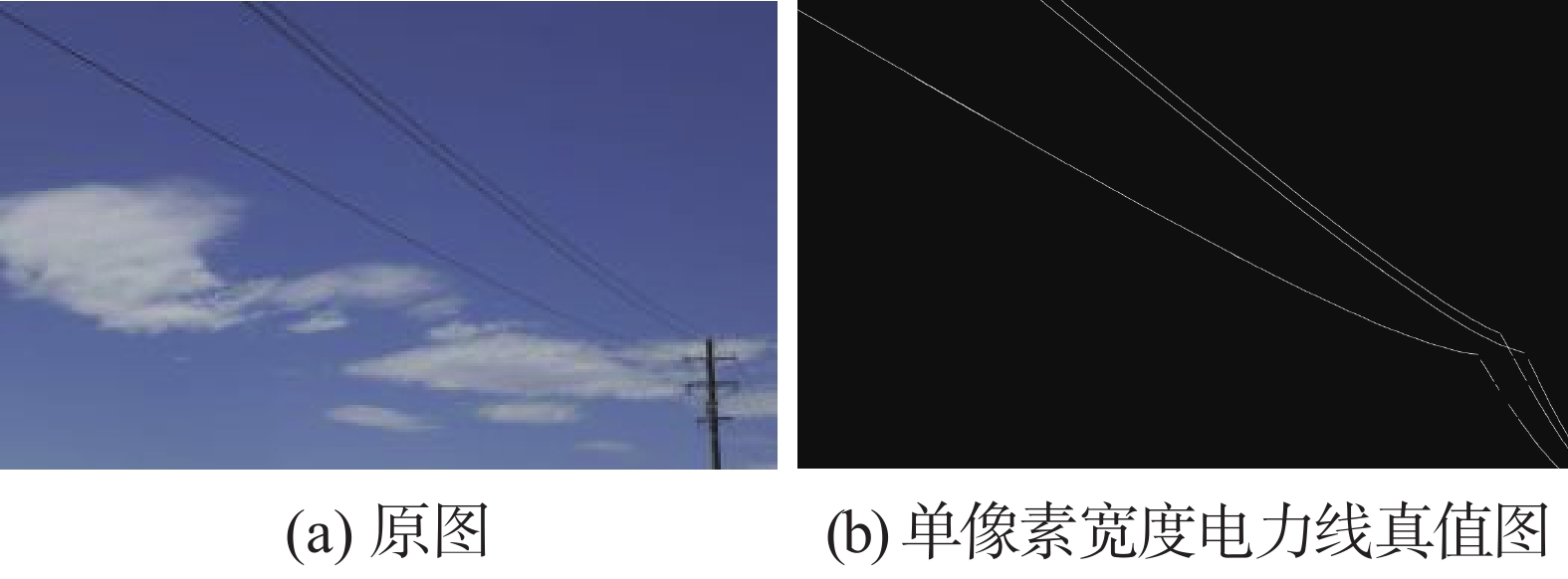Download: 图 10 电力线真值图表 1 检测精度定量分析

3 结论

  邓志远. 无人机在高压输电线路巡视中的应用研究[D]. 北京: 华北电力大学(北京), 2015. (0)  谢小伟, 彭向阳, 刘正军, 等. 无人直升机电力线路巡检自动航迹优化[J]. 测绘科学, 2015, 40(8): 87-91. (0)  李彩林, 冯朝晖, 邓晓景, 等. 复杂地物背景下的电力线提取方法[J]. 计算机工程与应用, 2016, 52(22): 198-202. DOI:10.3778/j.issn.1002-8331.1603-0446 (0)  徐青松, 季洪献, 王孟龙. 输电线路弧垂的实时监测[J]. 高电压技术, 2007, 33(7): 206-209. DOI:10.3969/j.issn.1003-6520.2007.07.047 (0)  谭金石, 郭明. 直升机电力巡检中电力线的自动提取[J]. 地理空间信息, 2012, 10(3): 70-71. DOI:10.3969/j.issn.1672-4623.2012.03.023 (0)  宋必芹. 光学影像中线结构提取技术及应用研究[D]. 西安:中国科学院研究生院(西安光学精密机械研究所), 2014. (0)  曲烽瑞, 董选昌, 李艳飞, 等. 基于多影像立体测量的电力线建模[J]. 自动化与仪器仪表, 2017(2): 13-14. (0)  VON GIOI R G, JAKUBOWICZ J, MOREL J M, et al. LSD: a fast line segment detector with a false detection control[J]. IEEE transactions on pattern analysis and machine intelligence, 2010, 32(4): 722-732. DOI:10.1109/TPAMI.2008.300 (0)  杨亮, 李辉芳, 江万寿, 等. 基于梯度对称性的无人机影像电力线自动提取方法[J]. 地理信息世界, 2017, 24(2): 94-99. DOI:10.3969/j.issn.1672-1586.2017.02.018 (0)  王宇, 王硕, 谭民. 基于LSD的具有特定特征的三维物体轮廓提取算法[J]. 系统科学与数学, 2016, 36(9): 1410-1416. (0)  段建民, 张云飞, 郑榜贵. 基于改进简单图像统计算法的车道线识别[J]. 计算机工程与设计, 2018, 39(3): 842-847. (0)  郭芸, 王宜怀, 刘纯平, 等. 基于主曲线的遥感图像河岸线提取[J]. 通信学报, 2016, 37(11): 80-89. DOI:10.11959/j.issn.1000-436x.2016222 (0)  李艳花. 浅析平面内两直线的位置关系[J]. 数学学习与研究, 2013(9): 97-98. (0)  王健一, 李金忠, 张书琦, 等. 大跨越输电线路载流量与弧垂模型[J]. 高电压技术, 2016, 42(13): 2974-2979. (0)  张永涛, 贾延明. 最小二乘法中代数多项式曲线拟合的分析及实现[J]. 计算机与数字工程, 2017, 45(4): 637-639, 654. DOI:10.3969/j.issn.1672-9722.2017.04.009 (0)  李慧鹏, 朱伟伟, 谭朦曦, 等. 基于改进Hough变换的直线检测算法[J]. 半导体光电, 2017, 38(4): 603-608. (0)